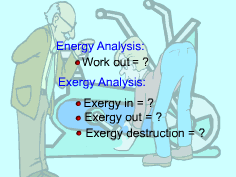Ch 7. Exergy Analysis Multimedia Engineering Thermodynamics Exergy ExergyChange ExergyBalance(1) ExergyBalance(2)
 Chapter 1. Basics 2. Pure Substances 3. First Law 4. Energy Analysis 5. Second Law 6. Entropy 7. Exergy Analysis 8. Gas Power Cyc 9. Brayton Cycle 10. Rankine Cycle Appendix Basic Math Units Thermo Tables Search eBooks Dynamics Fluids Math Mechanics Statics Thermodynamics Author(s): Meirong Huang Kurt Gramoll ©Kurt GramollTHERMODYNAMICS - CASE STUDY IntroductionProblem Description A parallel-shaft gearbox is planned to be installed on a new exercise machine. This gearbox receives work through a high-speed shaft and then delivers work through a low-speed shaft. The gearbox is cooled on its outer surface by convection with the surrounding air. A test is needed to exam the performance of this gearbox. What is known: The gearbox receives 60 kW through a high-speed shaft. The outer surface of the gearbox is 0.8 m2. Heat transfer coefficient between the gearbox and its surrounding air is 1.8 kW/m2-K Temperature of the outer surface of the gearbox is 40oC. The ambient pressure is 1 bar, and the ambient temperature is 25oC. Question Determine the work delivered by this gearbox. Explore the exergy transfer of the gearbox and the exergy destruction. Approach Take the gear box as a closed system. Use the energy balance of the gear box to determine the work output through the low-speed shaft. The exergy is transferred by heat and work. Exergy destruction is given as Xdestroyed = T0Sgen The entropy balance for the gearbox is: Sin - Sout + Sgen = ΔSsystem